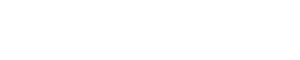# Electrical Machines – II

#### Course Topics

• THREE PHASE INDUCTION MOTOR
• CONSTRUCTION
• PRINCIPLE OF OPERATION
• EQUIVALENT CIRCUIT AND PERFORMANCE
• NO LOAD AND BLOCKED ROTOR TEST
• CIRCLE DIAGRAM
• LOSSES AND EFFICIENCY
• OPERATING CHARACTERISTICS AND INFLUENCE OF MACHINE PARAMETERS ON THE PERFORMANCE OF THE MOTOR
• STARTING, SPEED CONTROL AND BRAKING OF POLY -PHASE INDUCTION MOTORS
• STARTING OF SQUIRREL CAGE MOTORS
• STARTING OF WOUND ROTORS
• SPEED CONTROL OF THREE PHASE INDUCTION MOTOR
• ELECTRICAL BRAKING OF POLY PHASE INDUCTION MOTORS
• THE SINGLE PHASE INDUCTION MOTOR
• THEORIES OF OPERATION
• DOUBLE FIELD REVOLVING THEORY
• VOLTAGE EQUATION AND EQUIVALENT CIRCUIT
• DETERMINATION OF PARAMETERS BY TESTS
• SPLIT PHASE STARTING
• REPULSION MOTOR STARTING
• CAPACITOR MOTOR
• AC TACHOMETER.
• TWO PHASE CONTROL MOTOR, CROSS FIELD THEORY
• SPECIAL ELECTRICAL MACHINE
• INDUCTION VOLTAGE REGULATOR
• SYNCHRONOUS INDUCTION MOTOR
• POWER SYNCHROS ,POSITION SYNCHROS
• LINEAR MOTOR
• SYNCHRONOUS MACHINES
• ALTERNATORS OR THREE PHASE SYNCHRONOUS GENERATORS
• INTRODUCTIONS, CONSTRUCTIONAL FEATURES OF CYLINDRICAL AND SALIENT POLE ROTOR
• ARMATURE WIINDING
• CHORDING OF WINDINGS
• PITCH FACTOR